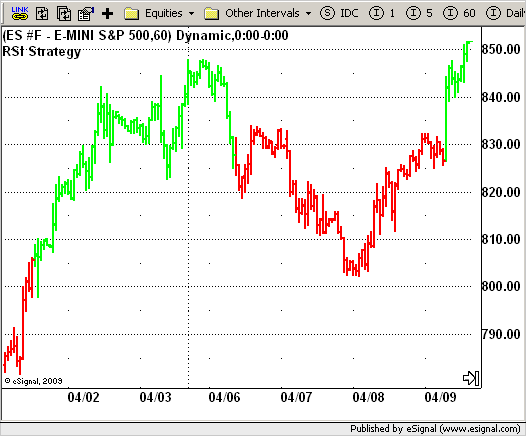# RSI Strategy

ICE Data Services -

strRSI.efs
EFSLibrary - Discussion Board

File Name: strRSI.efs

Description:
RSI Strategy

Formula Parameters:

• nLength : 5
• Overbought : 70
• Oversold : 30

Notes:

The RSI is a very popular indicator that follows price activity.
It calculates an average of the positive net changes, and an average of the negative net changes in the most recent bars, and it determines the ratio between these averages. The result is expressed as a number
between 0 and 100. Commonly it is said that if the RSI has a low value, for example 30 or under, the symbol is oversold. And if the RSI has a high value, 70 for example, the symbol is overbought.

strRSI.efsEFS Code:

```/*********************************
Provided By:
eSignal (Copyright c eSignal), a division of Interactive Data
Formula Script (EFS) is for educational purposes only and may be
modified and saved under a new file name.  eSignal is not responsible
for the functionality once modified.  eSignal reserves the right
to modify and overwrite this EFS file with each new release.

Description:
RSI Strategy

Version:            1.0  05/12/2009

Formula Parameters:                     Default:
nLength                             5
Overbought                          70
Oversold                            30

Notes:
The RSI is a very popular indicator that follows price activity.
It calculates an average of the positive net changes, and an average
of the negative net changes in the most recent bars, and it determines
the ratio between these averages. The result is expressed as a number
between 0 and 100. Commonly it is said that if the RSI has a low value,
for example 30 or under, the symbol is oversold. And if the RSI has a
high value, 70 for example, the symbol is overbought.
**********************************/
var fpArray = new Array();
var bInit = false;

function preMain() {
setPriceStudy(true);
setColorPriceBars(true);
setStudyTitle("RSI Strategy");
setDefaultPriceBarColor(Color.black);
var x = 0;
fpArray[x] = new FunctionParameter("nLength", FunctionParameter.NUMBER);
with(fpArray[x++]) {
setLowerLimit(1);
setDefault(12);
}
fpArray[x] = new FunctionParameter("Oversold", FunctionParameter.NUMBER);
with(fpArray[x++]) {
setLowerLimit(1);
setDefault(30);
}
fpArray[x] = new FunctionParameter("Overbought", FunctionParameter.NUMBER);
with(fpArray[x++]) {
setLowerLimit(1);
setDefault(70);
}
}

var xRSI = null;

function main(nLength, Oversold, Overbought) {
var nBarState = getBarState();
var nRSI = 0;
if (nBarState == BARSTATE_ALLBARS) {
if (nLength == null) nLength = 12;
if (Overbought == null)  Overbought = 70;
if (Oversold == null) Oversold = 30;
}
if (bInit == false) {
xRSI = rsi(nLength);
bInit = true;
}
if(getCurrentBarIndex() == 0) return;
nRSI = xRSI.getValue(0);
if(nRSI == null) return;
if(nRSI > Overbought && !Strategy.isLong())
Strategy.doLong("Long", Strategy.MARKET, Strategy.NEXTBAR);
if(nRSI < Oversold && !Strategy.isShort())
Strategy.doShort("Short", Strategy.MARKET, Strategy.NEXTBAR);
if(Strategy.isLong())
setPriceBarColor(Color.lime);
else if(Strategy.isShort())
setPriceBarColor(Color.red);
return;
}```